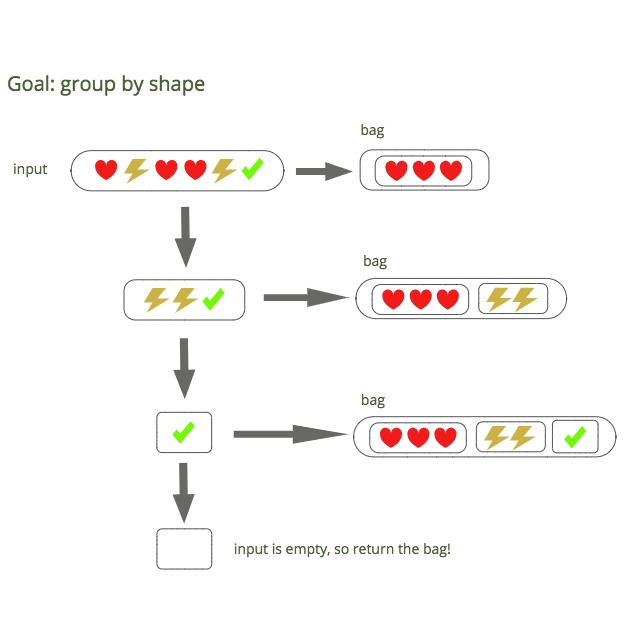#### Overview

One less understood idea among javascript engineers (unless you happen to be studying up for interviews) is the use of greedy algorithms. A greedy algorithm makes whatever choice seems best at the moment, and solves the subproblems that arise later. To use a visual metaphor, we put the result of each subproblem in a “bag” and then repeat with successively smaller subproblems. When the subproblem is empty (nothing left to do), we return the contents of the bag.

As it turns out, this strategy can lead to some very elegant solutions to practical problems. In the rest of this article, we’ll explore four seemingly different problems that have almost identical solutions (hint: they all use a greedy algorithm). In closing, we’ll take a closer look at the structure common to all four problems. Let’s dive in!

#### Example: coin change problem

Take a moment to consider how you’d do this before continuing… (answer is right below)

We keep a “bag” of coins and recursively add coins to the bag that matches our selection criteria `(pick largest coin denomination that is < amount)`. If the largest coin has value `C`, we add `C` to the bag and call `makeChange` with `amount - C`. This continues until the `amount` is 0, and the bag of coins is returned.

A quick note on the expression `{ ...bag, ...{ [fn(array)]: matches } }` since there’s a lot going on there. First of all, what does `{ ...a, ...b }` mean? This is called object spreading. Think of it as smooshing together objects a and b to create a new object. So `{ ...bag, ...somethingElse }` will combine the object `bag` with object `somethingElse`. In this case, `somethingElse` is the object `{ [fn(array)]: matches }` which is the new group we’re inserting into the bag.

I’ll also explain the difference between `{ [key]: value }` and `{ key: value }`. Those square braces signify computed properties. You can stick any expression between the square braces, and the value of that expression will become the value of the key. So for example `{ [1 + 1]: 2}` would be the same as `{ 2: 2 }`.

#### Example: groupBy

Take a moment to consider how you’d do this before continuing… (answer is right below)

Keep a “bag” of groups and recursively add groups to the bag that match our selection criteria `fn(x) === fn(array)`. Then call `groupBy` on the remaining elements, with the updated bag. This continues until the original array is empty, and the bag is returned.

#### Example: activity selection problem

Another classic problem is the activity selection problem.

Take a moment to consider how you’d do this before continuing… (answer is right below)

#### Example: collect anagrams

For our final example, we’ll consider the problem of grouping anagrams.

Take a moment to consider how you’d do this before continuing… (answer is right below)

#### The Common Structure

So what do all of these problems have in common? For every iteration through the loop, we select a subset of items from the input and add it to the bag. The remaining items feed through to the next iteration of the loop as the next input. When the input is empty, we return the bag.

The following diagram might help clarify things, using our groupBy example:If you’re more comfortable with pseudocode, here is the pattern we used in all of the previous examples:

#### Connect

What do you think? Have you solved similar problems at work or personal projects using greedy algorithms? Let me know in the comments below, or on twitter!